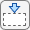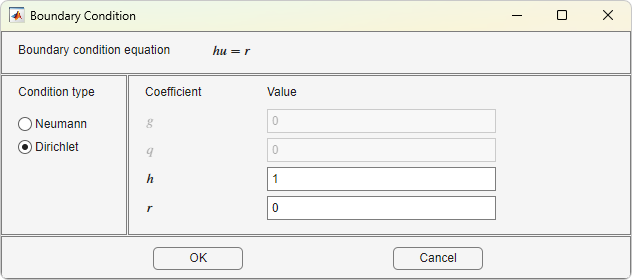## Specify Boundary Conditions in the PDE Modeler App

Select Boundary Mode from the Boundary menu or click thebutton. Then select a boundary or multiple boundaries for which you are specifying the conditions. Note that no if you do not select any boundaries, then the specified conditions apply to all boundaries.

• To select a single boundary, click it using the left mouse button.

• To select several boundaries and to deselect them, use Shift+click (or click using the middle mouse button).

• To select all boundaries, use the Select All option from the Edit menu.

Select Specify Boundary Conditions from the Boundary menu.Specify Boundary Conditions opens a dialog box where you can specify the boundary condition for the selected boundary segments. There are three different condition types:

• Generalized Neumann conditions, where the boundary condition is determined by the coefficients `q` and `g` according to the following equation:

`$\stackrel{\to }{n}\text{\hspace{0.17em}}·\text{\hspace{0.17em}}\left(c\nabla u\right)+qu=g.$`

In the system cases, `q` is a 2-by-2 matrix and `g` is a 2-by-1 vector.

• Dirichlet conditions: u is specified on the boundary. The boundary condition equation is hu = r, where h is a weight factor that can be applied (normally 1).

In the system cases, `h` is a 2-by-2 matrix and `r` is a 2-by-1 vector.

• Mixed boundary conditions (system cases only), which is a mix of Dirichlet and Neumann conditions. `q` is a 2-by-2 matrix, `g` is a 2-by-1 vector, `h` is a 1-by-2 vector, and `r` is a scalar.

The following figure shows the dialog box for the generic system PDE (Options > Application > Generic System).For boundary condition entries you can use the following variables in a valid MATLAB® expression:

• The 2-D coordinates `x` and `y`.

• A boundary segment parameter `s`, proportional to arc length. `s` is 0 at the start of the boundary segment and increases to 1 along the boundary segment in the direction indicated by the arrow.

• The outward normal vector components `nx` and `ny`. If you need the tangential vector, it can be expressed using `nx` and `ny` since tx = –ny and ty = nx.

• The solution `u`.

• The time `t`.

Note

If the boundary condition is a function of the solution `u`, you must use the nonlinear solver. If the boundary condition is a function of the time t, you must choose a parabolic or hyperbolic PDE.

Examples: `(100-80*s).*nx`, and `cos(x.^2)`

In the nongeneric application modes, the Description column contains descriptions of the physical interpretation of the boundary condition parameters.

## Support